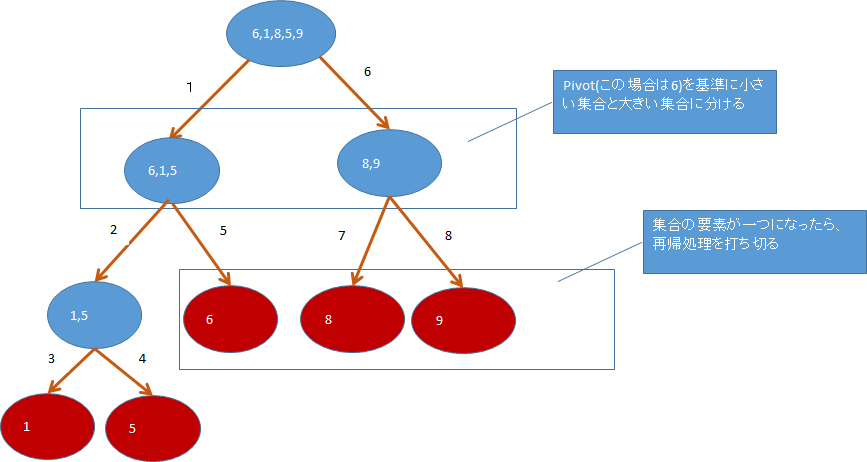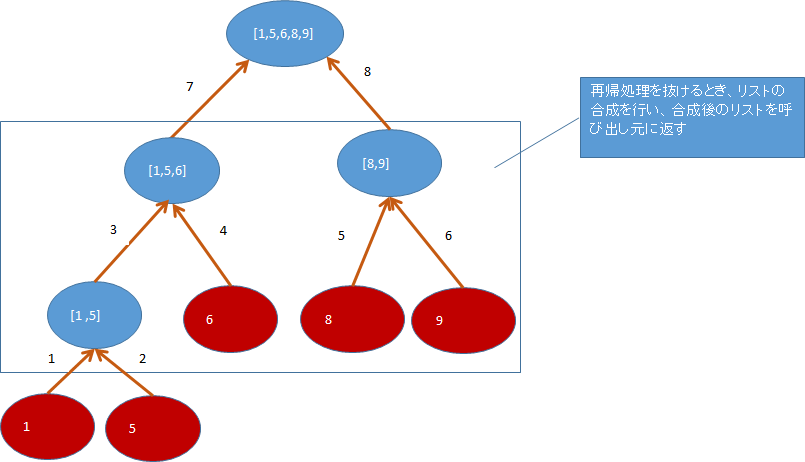# At the beginning

Since the implementation of quicksort explained on the net did not come well, I will upload an article that I summarized myself. The algorithm policy adopts element decomposition and reaggregation, which is often taken up in the explanation of functional languages, and I will try to implement it in Java and see how troublesome it is to implement quicksort in imperative languages.

# The idea of quicksort

As an example, consider sorting a set of numbers [6,1,8,5,9]. When doing quicksort, we need a criterion to divide the elements, but here we will use the first element of the set as the criterion.

## Disassembled image of the list## Image of aggregated list after decomposition# Implementation code

#### `Qsort.java`

``````
package test;

import java.util.ArrayDeque;
import java.util.ArrayList;
import java.util.Arrays;
import java.util.Deque;
import java.util.List;

public class QSort {

public static void main(String args[]){
System.out.println(qsort(Arrays.asList(1,5,6,2,11,9,4)));
}

public static List<Integer> qsort(List<Integer>list){
if(list.size()==1){
//If the array contains one element, exit the recursive process
return new ArrayList<Integer>(list) ;
}else{
//Call the process to split the elements in the list
Divive div = splitList(list);
//Generate a list to store the split array
List<Integer>newList = new ArrayList<Integer>();
//Perform recursive processing to separate a small set of numbers again
//Perform recursive processing to isolate a large set again
return newList ;
}
}

//Function to split the list
public static Divive splitList(List<Integer>list){
int size =list.size();
Divive div = new Divive();
//When the number of elements is two, the size of the elements is compared and the elements are divided.
if(size==2){
if(list.get(0)<=list.get(1)){
}else{
}
return div;
}

int pivot = list.get(0);
List<Integer>smallIntList =new ArrayList<Integer>();
List<Integer>largeIntList =new ArrayList<Integer>();
//Divide the list given by the argument into a small set and a large set according to a predetermined criterion.
for(int i=0;i<size;i++){
//Generate a set of numbers smaller than the reference
//Generate a set of numbers larger than the reference
if(pivot<list.get(size - 1- i))largeIntList.add(list.get(size - 1- i));
}

//If the arguments of the list given in the argument do not match the small set, return the split list combination to the caller.
if(smallIntList.size()!=list.size()){
}
//If the argument of the list given by the argument matches the small set, the reference number is the smallest, so
//Split the list on the far left of the list and elsewhere
else{
Deque<Integer> que  = new ArrayDeque<Integer>(smallIntList);